# Calculus 2 : Graphing Vectors

## Example Questions

### Example Question #31 : Parametric, Polar, And Vector Functions

The graph of the vector function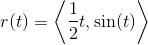can also be represented by the graph of which of the following functions in rectangular form?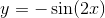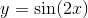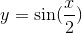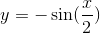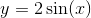Explanation:

We can find the graph of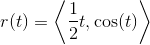in rectangular form by mapping the parametric coordinates to Cartesian coordinates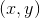: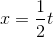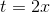We can now use this value to solve for: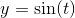### Example Question #12 : Graphing Vectors

The graph of the vector function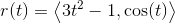can also be represented by the graph of which of the following functions in rectangular form?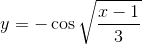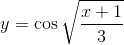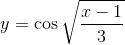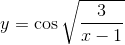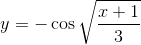Explanation:

We can find the graph ofin rectangular form by mapping the parametric coordinates to Cartesian coordinates: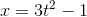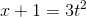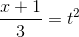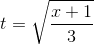We can now use this value to solve for:### Example Question #11 : Graphing Vectors

The graph of the vector function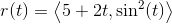can also be represented by the graph of which of the following functions in rectangular form?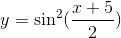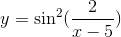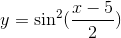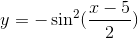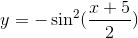Explanation:

We can find the graph ofin rectangular form by mapping the parametric coordinates to Cartesian coordinates: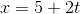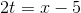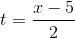We can now use this value to solve for: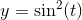### Example Question #12 : Graphing Vectors

In which quadrant does the vector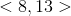terminate from the origin?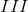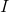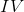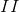Explanation:

The vectorgoesunits right from the origin andunits up, terminating in Quadrant I.

### Example Question #13 : Graphing Vectors

In which quadrant does the vector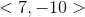terminate from the origin?Explanation:

The vectorgoesunits right from the origin andunits down, terminating in Quadrant IV.

### Example Question #14 : Graphing Vectors

In which quadrant does the vector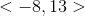terminate from the origin?Explanation:

The vectorgoesunits left from the origin andunits up, terminating in Quadrant II.

### Example Question #15 : Graphing Vectors

In which quadrant does the vector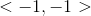terminate from the origin?Explanation:

The vectorgoesunit left from the origin andunits down, terminating in Quadrant III.

### Example Question #12 : Graphing Vectors

In which quadrant does the vectorterminate from the origin?

Explanation:

From the origin, the vectorgoes to the rightunits and upunits, terminating in

### Example Question #13 : Graphing Vectors

In which quadrant does the vector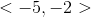terminate from the origin?

Explanation:

From the origin, the vectorgoes to the leftunits and downunits, terminating in

### Example Question #14 : Graphing Vectors

In which quadrant does the vector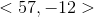terminate from the origin?

From the origin, the vectorgoes to the right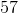units and downunits, terminating in